Electric

This chapter is about current and potential difference, resistance, electromotive force (e.m.f) and internal resistance and also electrical energy and power.

 3.1 Current and Potential Difference

 Electric field The region around a charged particle where any electric charge in the region will experience an electric force

The patterns of observed electric fields for positive charge and negative charge are as follows:

 (a)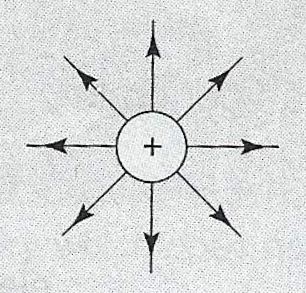The direction of the electric force line avoids the positive charge. (b)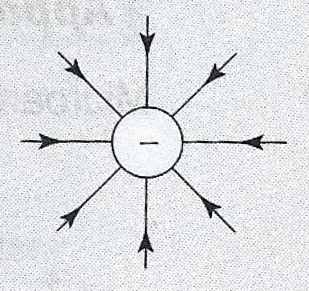The direction of the electric force line to negative charge.

 Electric field strength, E Electric force acting on a unit positive charge placed at the point. $$E=\dfrac{F}{q}$$, where E = electric field strength, F = electric force, Q = quantity of electric charge $$E=\dfrac{V}{d}$$, where E = electric field strength, V = potential difference, d = distance between plates

 Electric current, $$I$$ Rate of flow of charge in a conductor $$I = \dfrac{Q}{t}$$, $$I$$ = current, Q = total charge, t = time

 Potential difference Work done in moving one coulomb of charge from one point to another. $$V = \dfrac{W}{Q}$$ or $$V=\dfrac{E}{Q}$$, where V= potential difference, W = work done,  E = energy transferred, Q = amount of charges flowing

 3.2 Resistance

 Ohm's law Potential difference flowing through a conductor is directly proportional to the electric current when the temperature and other physical properties are kept constant. $$V = I\times R$$

If Ohm's law is obeyed, the graph against it or otherwise is a straight line as follows: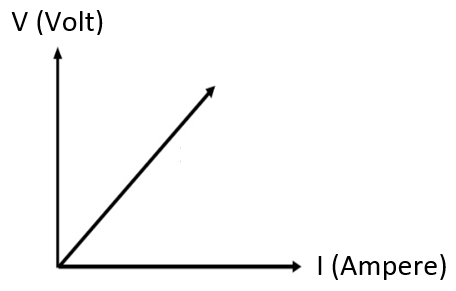Ohmic conductor Conductor which obeys Ohm's Law (Resistance constant) Example: Constantan wire

 Non-ohmic conductor Conductor which does not obeys Ohm's Law (Resistance constant) Example: Filament bulb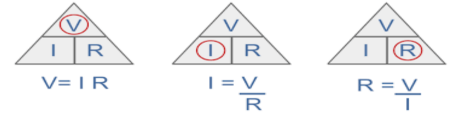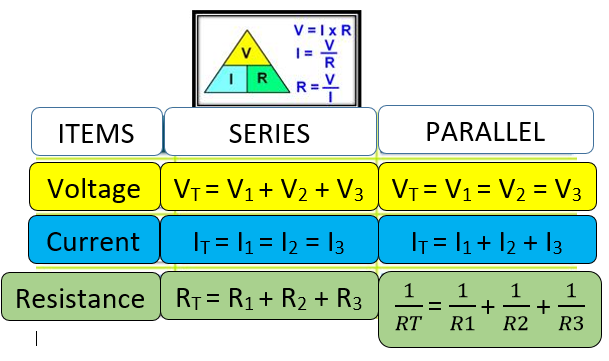Resistance of wire $$R=\dfrac{\rho\,l}{A}$$

Factors that affect the resistance of a wire

• Length of wire, $$l$$
• $$l$$ increase, $$R$$ increase
• cross sectional area of wire, $$A$$
• $$A$$ increase, $$R$$ decrease
• resistivity of the wire, $$\rho$$
• $$\rho$$ increase, $$R$$ increase

 Resistivity of a conductor, $$\rho$$ a measure of a conductor's ability to oppose the flow of electric current unit is ohm-meter depends on the temperature and the nature of the conductor material

 3.3 Electromotive Force (e.m.f) and Internal Resistance

 Electromotive force, $$\varepsilon$$ Energy transferred or work done by an electrical source to move one coulomb of charge in a complete circuit. $$\varepsilon=\dfrac{E}{Q}$$, where, $$\varepsilon$$ = electromotive force, E = energy transferred / work done, Q = the amount of charge flowing

 Internal resistance, r Resistance caused by electrolyte in the dry cell. $$\varepsilon > V$$ $$Ir = \varepsilon - V$$

 Formula relating E, V, I, R and r $$\varepsilon = V+Ir$$ $$\varepsilon = I(R+r)$$

 3.4 Electrical Energy and Power

 Relationship between$$E\,,V\,,I$$ and $$t$$ $$E = V \,I\, t$$

 Relationship between$$P\,,V$$ and $$I$$ $$P=V\,I$$

 Relationship between$$P\,,V\,,I$$ and $$R$$ $$P=I^2\,R$$ $$P=\dfrac{V^2}{R}$$

 Energy consumed for electrical devices, $$E$$ $$E = P\,t$$

 Cost of consumption $$\text{Total cost} = E \times \text{Cost per kWh}$$